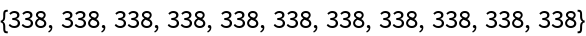#Function Repository Resource:

# PartitionCrank

Get Dyson's crank of an integer partition

Contributed by: George Beck
 ResourceFunction["PartitionCrank"][x] gives Dyson's crank of the partition x.

## Details and Options

A partition of a positive integer n is a weakly decreasing list of positive integers that add up to to n.
The crank is a partition statistic useful in studying congruence properties of the partition function p(n), given by PartitionsP in the Wolfram Language.

## Examples

### Basic Examples

Here is a basic example:

 In:=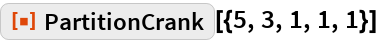Out=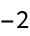Here are the partitions of 9 in compact form:

 In:=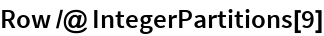Out=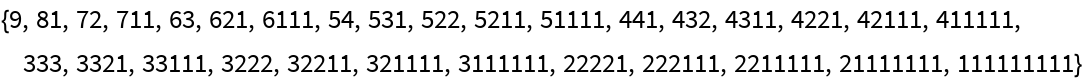The crank of the partitions of 9:

 In:=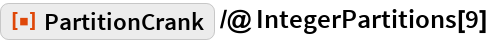Out=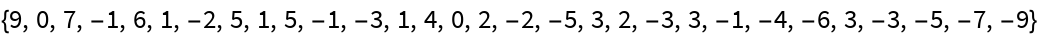### Neat Examples

Ramanujan discovered and proved analytically that the number of partitions of 11m+6 is divisible by 11:

 In:=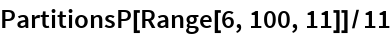Out=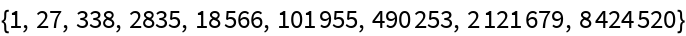Andrews and Garvan proved that the partitions of a number of the form 11m+6 can be split into 11 sets of equal size according to the crank mod 11:

 In:=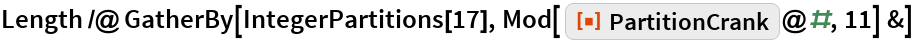Out=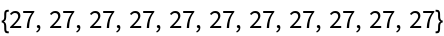Here is an example using a different value of m:

 In:=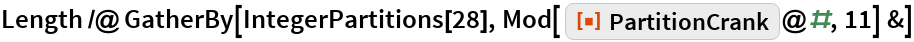Out=